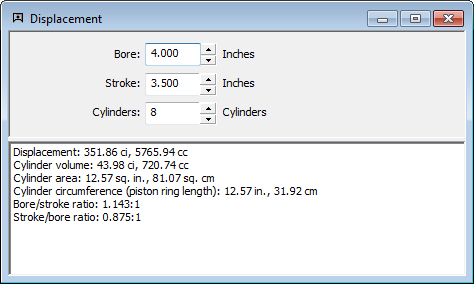## Displacement Calculation

• Calculates engine displacement
• Calculates cylinder volume
• Calculates cylinder area
• Calculates piston ring length
• Calculates bore/stroke and stroke/bore ratioEnter your engine's bore, stroke, and number of cylinders. Calculate displacement, cylinder volume, cylinder area, cylinder circumference, piston ring length, bore/stroke ratio, and stroke/bore ratio.